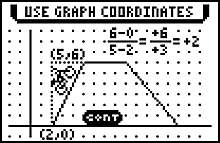# Software, OS updates and apps

## Topics in Algebra 1 (Chapters 1-5) App for TI-73 Explorer™The power of an Algebra 1 tutorial on your calculator.

Topics in Algebra: Chapter 1 - Number Sense 1.01 72 2
Topics in Algebra: Chapter 5 - Linear Systems 1.00 76 2
Topics in Algebra: Chapter 2 - Linear Equations 1.01 72 2
Topics in Algebra: Chapter 3 - Linear Functions 1.01 77 2
Topics in Algebra: Chapter 4 - Linear Inequalities: 1-Variable 1.01 72 2
Guidebooks
Topics in Algebra I guidebook for TI-83 Plus / TI-84 Plus (English) View: 2,484

## Features Summary

The power of an Algebra 1 tutorial on your calculator. Students can practice basic Algebra 1 concepts using the tutorial and practice activities to develop algebra comprehension.

Students can explore concepts such as:

• Number sense
• Linear equations
• Linear functions
• Linear inequalities

Students are also able to:

• See real problems that are modeled using linear systems
• Investigate the solution to linear systems using graphs and tables
• Review how to solve linear systems using the algebraic methods of substitution and elimination

In addition, students can download and use the worksheets below to view a step-by-step visual representation of critical concepts, then complete activities to reinforce each concept.

The Topics in Algebra I App is available for the TI-73 Explorer™, TI-83 Plus, TI-83 Plus Silver Edition, TI-84 Plus, and TI-84 Plus Silver Edition Graphing Calculators.